# Mathematics - Signals And Systems - Exercises on System Representation using Difference Equations

in StemSocial3 months ago[Image1]

## Introduction

Hey it's a me again @drifter1!

Today we continue with my mathematics series about Signals and Systems in order to cover Exercises on System Representation using Difference Equations.

So, without further ado, let's dive straight into it!

## Difference Equation Representation

In an previous article of the series it was explained how discrete-time LTI systems can be represented using linear constant-coefficient difference equations (LCCDE), which are of the form: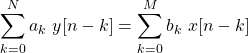This representation led to us to very useful Block Diagram Representations, such as the Direct Form I and II Implementations.

## Diagram to Equation [Based on P6.4 from Ref1]

Let's consider the following block diagram, which relates the signals x[n] and y[n]: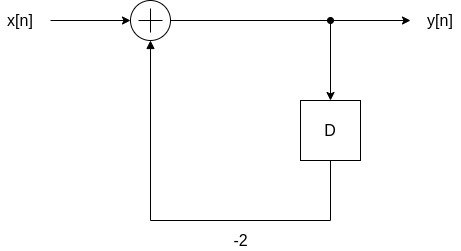Assuming causality and that the system is initially at rest:

1. Determine the LCCDE that relates y[n] and x[n]
2. Determine the value of y[-3] for x[n] = u[n]
3. Determine the solution of the LCCDE for x[n] = δ[n]
4. Determine the value of y, y and y for x[n] = δ[n]

### a.

In the block diagram y[n] gets delayed by one sample resulting in y[n - 1]. On this delayed sample of y[n] a gain of -2 is applied. Thus, the second input to the sum is -2 y[n - 1]. As simple as that, we now have all the information that we need to relate the two signals.

Let's label the diagram to understand this better.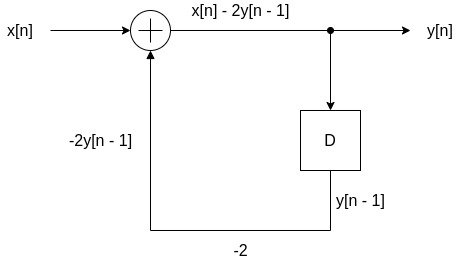So, in order to represent this simple system using a LCCDE, we simple have to write: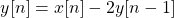### b.

Being causal, the output y[n] of the system is zero before a non-zero input is applied. In the case of u[n], this is true for n < 0, which leads to the conclusion that y[n] must also be zero for n < 0. Therefore, y[-3] = 0.

### c.

In order to calculate the other values the solution to the LCCDE is needed. In the case of this simple system, the solution is known to be of the form: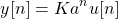Recalling that x[n] = y[n] = 0, n < 0, its easy to find the initial value of the output: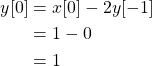where x = 1 because x[n] = δ[n], the unit pulse.

Re-writting the previous equation, and substituting y[n] with the solution form, its easy to find the correct K: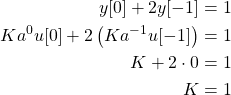Now for the parameter a. Knowing K, for n > 0, we now have: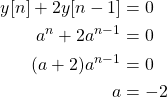So, the final solution of the LCCDE is: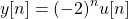As such, the left-hand side can now be re-written as: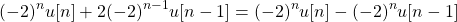which is equal to 1 only for n = 0 and zero otherwise.

### d.

The the system can be re-written as: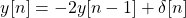Due to causality y[-1] = 0, and so we can directly calculate y, y, y: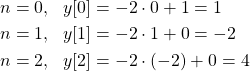The same answer can also be taken from the solution proven in c., as follows: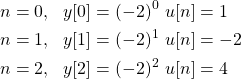Note that u[n] = 1, n >= 0.

## Direct Form to Equation [Based on P6.5 | P6.7 from Ref1]

Let's consider the following Direct Form II implementation of a difference equation that describes an LTI system: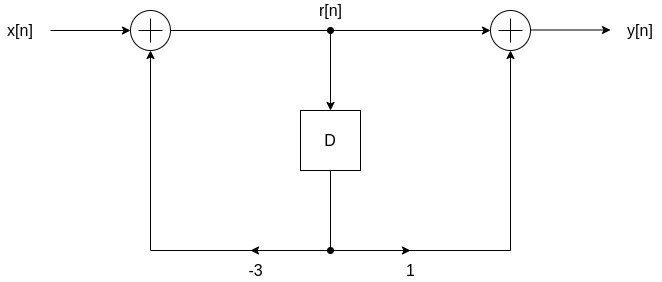Let's determine the following:

1. Direct Form I implementation
2. Difference Equation that describes the system using the:
• Direct Form I implementation
• Direct Form II implementation

### a.

The Direct Form II implementation is a somewhat compressed version of Form I, where the delay units are shared. As such, converting from Form II to I, and vise versa, is quite simple.

More specifically, the Direct Form I implementation is: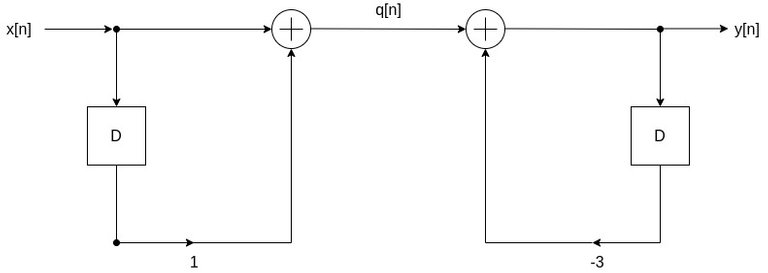### b.

Let's first continue on from the Direct Form I representation in order to get the LCCDE representation of the system.

From the block diagram: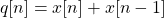and: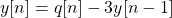Substituting for q[n] gives us the final representation: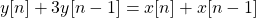This was quite simple, but how about Direct Form II?

In order to answer this question we have to find out how the intermediate signal r[n] is related to x[n] and y[n], respectively. Using these relations it will be easy to verify that the relation of x[n] and y[n], and so the LCCDE, is the same in both cases.

From the block diagram, the intermediate signal is given by: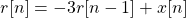which allows us to relate r[n] and x[n].

By recursively solving for x[n] = δ[n], we can find the signal that corresponds to r[n]: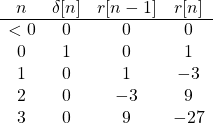Seeing how r[n] changes with the value of n, we are clearly talking about an exponential of -3. So, r[n] is defined as: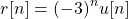From the block diagram, y[n] is related to r[n] as follows: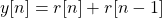For an input that is the unit pulse δ[n] the output is the impulse response h[n] of the system, and so: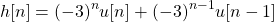For n < 0 this equation gives us zero, whilst for n = 0 it gives us 1. In the case of n > 0 after some calculations we will get: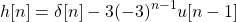And so, the relation of x[n] = δ[n] and y[n] = h[n] is the same as the relation calculated using Direct Form I: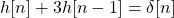## RESOURCES:

### Images

Block diagrams and other visualizations were made using draw.io

## Final words | Next up

And this is actually it for today's post!

Next time we will get into exercises on the Representation using Differential Equations!

See Ya!Keep on drifting!

Sort:Thanks for your contribution to the STEMsocial community. Feel free to join us on discord to get to know the rest of us!

Please consider supporting our funding proposal, approving our witness (@stem.witness) or delegating to the @stemsocial account (for some ROI).

Please consider using the STEMsocial app app and including @stemsocial as a beneficiary to get a stronger support.